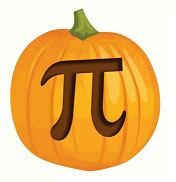### D - Digit sequence

##### Languages: C, C++, Java, Python, ... (details)

Fito is passioned about the conjecture that all natural numbers appear in the infinite digit sequence of $\pi = 3.14159265....$, therefore, every time he sees a sequence of digits, he tries to identify the natural numbers whose decimal representations appear as subsequences (in consecutive positions).For example, the digit sequence $1\ 0\ 2$ contains the natural numbers $1$, $2$, $10$ and $102$. Fito wants you to help him write a program that calculates, given a sequence of digits, which is the smallest natural number that does not appear as a subsequence. In the example above the answer would be $3$.

#### Input

The input consists of a single test case that contains a line with the digit sequence (at most $1000$ digits).

#### Output

Print the smallest natural number that does not appear in the sequence.

Input
102
Output
3
Input
56810374902
Output
11